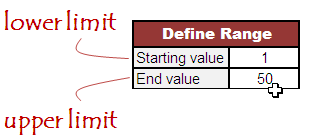# How to generate unique random numbers in Excel

This article shows how to generate unique random numbers in an interval in Excel.

Microsoft Excel includes a built-in function called RANDBETWEEN that generates a random number within a specified range. However, the numbers generated by this function can sometimes repeat. In this article, we will cover two different methods to ensure that a list of random numbers does not contain any duplicates.

Method 1 : Rank random integers

Follow the steps below to generate non-repeating random numbers between 1 and 50.

1. Enter the RAND() function in cells B6 to B55.
2. In cell D6, input the formula =RANK(B6,B\$6:B\$55), and drag it down to cell D55.
Method 2 : Array Formula

Specify lower limit i.e. 1 in cell J3 and upper limit i.e. 50 in cell J4.Copy the following formula into cell F6 and paste it down :

`=IFERROR(LARGE(ROW(INDIRECT(\$J\$3&":"&\$J\$4))*NOT(COUNTIF(\$F\$5:F5,ROW(INDIRECT(\$J\$3&":"&\$J\$4)))),RANDBETWEEN(1,\$J\$4-\$J\$3-ROW(A1)+2)),"")`
Hit CTRL+ SHIFT + ENTER to confirm this formula. If done correctly, Excel will automatically place curly braces {...} around the formula.
How does the formula work?
1. The formula uses the INDIRECT function along with cell references J3 and J4 to create a dynamic range.
2. The ROW function generates an array of numbers corresponding to the range created in step 1.
3. The COUNTIF function checks if each number in the array already exists in the cells above in column F.
4. The NOT function inverts the results of the COUNTIF function.
5. The product of the array and the inverted results is passed to the LARGE function.
6. The LARGE function returns the kth largest value from the array, where k is the position of the number in the sorted array.
7. If an error occurs, the formula falls back to the RANDBETWEEN function to generate a random number.
How to modify the formula

Suppose you want 100 unique numbers between 5 and 105.

1. Enter the lower limit, 5, in cell J3 and the upper limit, 105, in cell J4.
2. In column F, copy the formula down to row F105.
Related Posts
ShareDeepanshu founded ListenData with a simple objective - Make analytics easy to understand and follow. He has over 10 years of experience in data science. During his tenure, he worked with global clients in various domains like Banking, Insurance, Private Equity, Telecom and HR.

4 Responses to "How to generate unique random numbers in Excel"
1.thank you so much

2.Thank you, saved me some headache time.

3.4.I tried the second method in Excel 2003, but the iferror function is not available there. Have you got an equivalent for Excel 2003?

Next → ← Prev